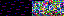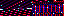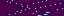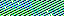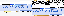```<?php
namespace entities\evr\security;

class Registrar
{
public function register()
{
\$this->set_join_flag();
{
if (Security::validate_key())
{
Security::remove_key();
return true;
}
}
return false;
}
{
{
\$name = null;
}
{
Security::show_error("Please use only a-z, A-Z, 0-9, or _");
\$name = null;
}
if (Security::find_user_directory(\$name))
{
\$name = null;
}
}
{
\$min = \$GLOBALS["USER_NAME_MIN_LENGTH"];
\$max = \$GLOBALS["USER_NAME_MAX_LENGTH"];
return \$this->check_length(\$name, \$min, \$max);
}
private function check_length(\$string, \$min, \$max)
{
\$length = strlen(\$string);
return \$length >= \$min && \$length <= \$max;
}
{
return preg_match("/^[a-zA-Z0-9_]*\$/", \$name);
}
{
{
}
{
}
{
}
}
{
}
{
\$chars = "/[a-zA-Z]/";
\$numerals = "/[0-9]/";
}
{
if (!preg_match("/.+@.+\..+/", \$email))
{
\$email = null;
}
\$this->email = \$email;
}
private function set_join_flag()
{
if (Security::get_post_parameter("join"))
{
\$this->join = "1";
}
else
{
\$this->join = "0";
}
}
{
\$this->create_user_directory();
\$this->create_history_file();
\$this->create_expert_file();
\$this->initialize_progress_file();
}
private function create_user_directory()
{
mkdir(\$this->get_user_path(), 0770);
}
{
\$path = \$this->create_file(\$GLOBALS["USER_HASH_PATH"]);
file_put_contents(\$path, \$hash . "\n");
}
private function create_file(\$name)
{
\$path = \$this->get_user_path() . \$name;
touch(\$path);
chmod(\$path, octdec(\$GLOBALS["USER_FILE_PERMISSIONS"]));
return \$path;
}
private function get_user_path()
{
return \$GLOBALS["USERS_PATH"] . "/" . \$this->username . "/";
}
{
\$salt = \$this->generate_salt();
}
private function generate_salt()
{
return chr(rand(65, 90)) . rand(0, 9);
}
{
\$content = \$this->email . " " . \$this->join . "\n";
file_put_contents(\$path, \$content);
}
{
}
private function create_history_file()
{
\$this->create_file(\$GLOBALS["USER_HISTORY_PATH"]);
}
private function create_expert_file()
{
\$this->create_file(\$GLOBALS["USER_EXPERT_PROGRESS_PATH"]);
}
private function initialize_progress_file()
{
\$path = \$this->create_file(\$GLOBALS["USER_PROGRESS_PATH"]);
file_put_contents(\$path, "0\n");
}
{
{
{
return true;
}
}
return false;
}
{
{
}
return false;
}
{
\$set = \$this->generate_character_set();
for (\$ii = 0; \$ii < \$length; \$ii++)
{
\$password .= \$set[rand(0, strlen(\$set) - 1)];
}
}
private function generate_character_set()
{
\$set = "";
for (\$ii = 0; \$ii <= 90 - 65; \$ii++)
{
\$set .= chr(\$ii + 65);
}
for (\$ii = 0; \$ii <= 122 - 97; \$ii++)
{
\$set .= chr(\$ii + 97);
}
for (\$ii = 0; \$ii <= 9; \$ii++)
{
\$set .= \$ii;
}
return \$set;
}
{
if (\$mail->send())
{
return true;
}
Security::show_error("Mail delivery failed");
return false;
}
{
\$fields = explode(" ", file_get_contents(\$path, FILE_IGNORE_NEW_LINES));
return \$fields;
}
}
```
3.215.182.36
3.215.182.36
3.215.182.36

November 9, 2013Food Spring - Watermelon Stage

Getting the fruit as far as possible is the object of each level, collecting bigger, more valuable guns. The final result is determined by the size of the fruits' collection when the monkey arrives in North America and either survives or perishes in the fruits' attack.Watermelon Peach Pineapple Grapes